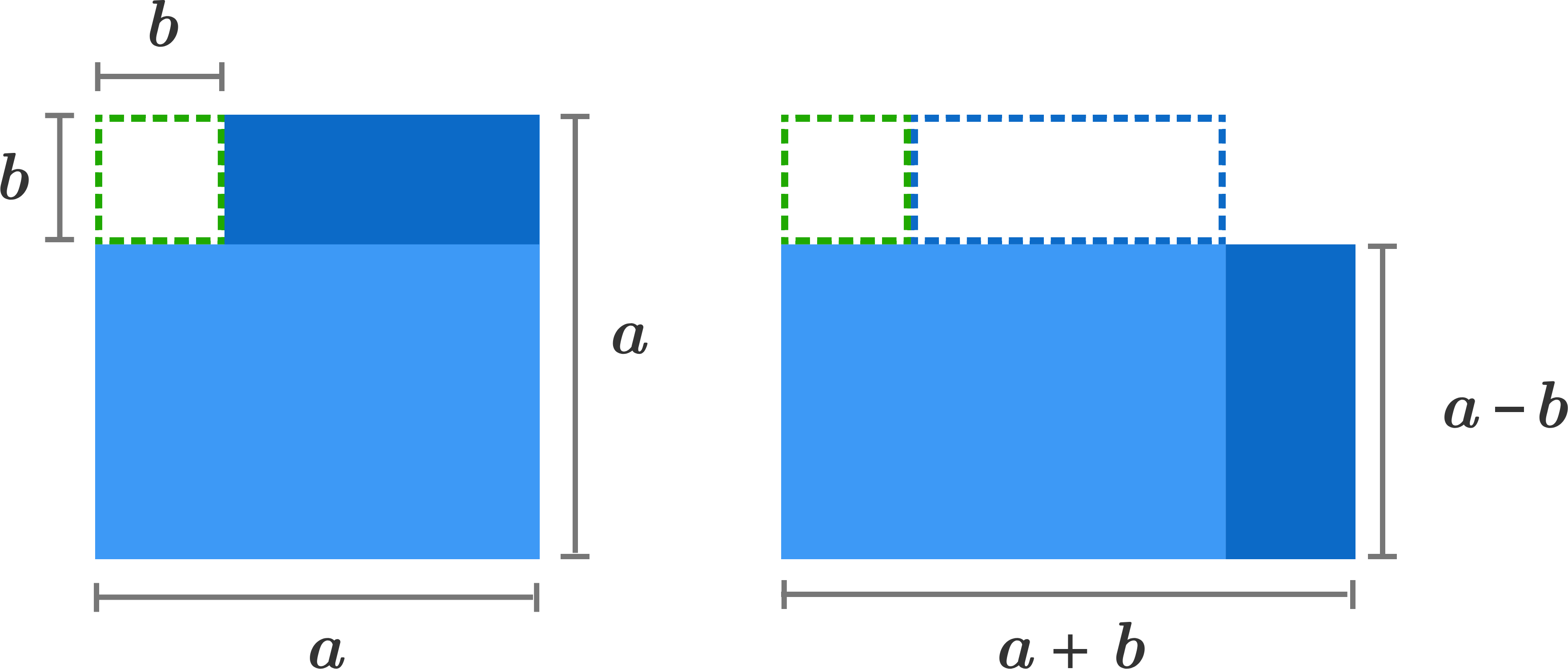Algebra I

Exponents allow us to write large numbers concisely. For example, $6 \times 6 \times 6 \times 6 \times 6 \times 6 \times 6 = 279,936$ but using exponents, we can write this simply as $6^7$.

While exponents make writing these values easier, it is often challenging or tedious to perform operations like addition and multiplication with such large numbers. In the upcoming problems, we'll see how the properties of exponents allow us to solve problems quickly and without needing to expand the exponential expressions.

Try to think of ways to solve the problems in this quiz that don't require expanding the exponents.

Exponent Shortcuts

Using the rules of exponents, simplify $\large \frac{ {\color{#20A900}{2}} ^ {\color{#3D99F6}{3}} \times {\color{#20A900}{2}} ^ {\color{#3D99F6}{7}}}{ {\color{#20A900}{2}} ^ {\color{#3D99F6}{6}} \times {\color{#20A900}{2}} ^ {\color{#3D99F6}{3}} }.$

Remember: you are not a robot, and you don't need a calculator to solve this!Exponent Shortcuts

In the last problem, we used exponent properties to simplify an expression. We can use the same properties to compare two values.

Which is larger,

$\large 3 ^ { 4 } + 3 ^ { 4 } + 3 ^ { 4 } \hspace{.5cm} \text{ or } \hspace{.55cm} {3} ^ { 5} \, ?$

Exponent Shortcuts

Evaluate

$\large \frac{ \color{#3D99F6}{42} ^ 2 - \color{#20A900}{38} ^ 2 } { \color{#3D99F6}{42} - \color{#20A900}{38} }.$

Hint: Use the difference of two squares identity: $a^2-b^2=(a+b)(a-b)$.Exponent Shortcuts

What is the value of $\LARGE {\color{#3D99F6}{ 2}} ^ { {\color{#20A900}{8}} ^{ {\color{#3D99F6} { 2} }} } ?$

Exponent Shortcuts

At which step is the first error made in solving the equation given in Step 1?

Step 1. $\,\,\,\sqrt{x + 4} = 5$

Step 2. $\,\,\,\sqrt{x} + \sqrt{4} = 5$

Step 3. $\,\,\,\sqrt{x} + 2 = 5$

Step 4. $\,\,\,\sqrt{x} = 3$

Step 5. $\,\,\,x = 9$

×